# R S Aggarwal Solutions for Class 10 Maths Chapter 4 Quadratic Equations Exercise 4C

The set of R S Aggarwal Solutions is a useful study material for students to practice on various Maths concepts and clear their doubts. Students can download free pdf of class 10 Maths Chapter 4 Quadratic Equations exercise 4C by clicking on the given link below. This exercise concept helps students solve quadratic equations using the quadratic formula which is also known as Shridharacharya’s Rule and nature of quadratic equations roots using discriminant.

## Download PDF of R S Aggarwal Solutions for Class 10 Maths Chapter 4 Quadratic Equations Exercise 4C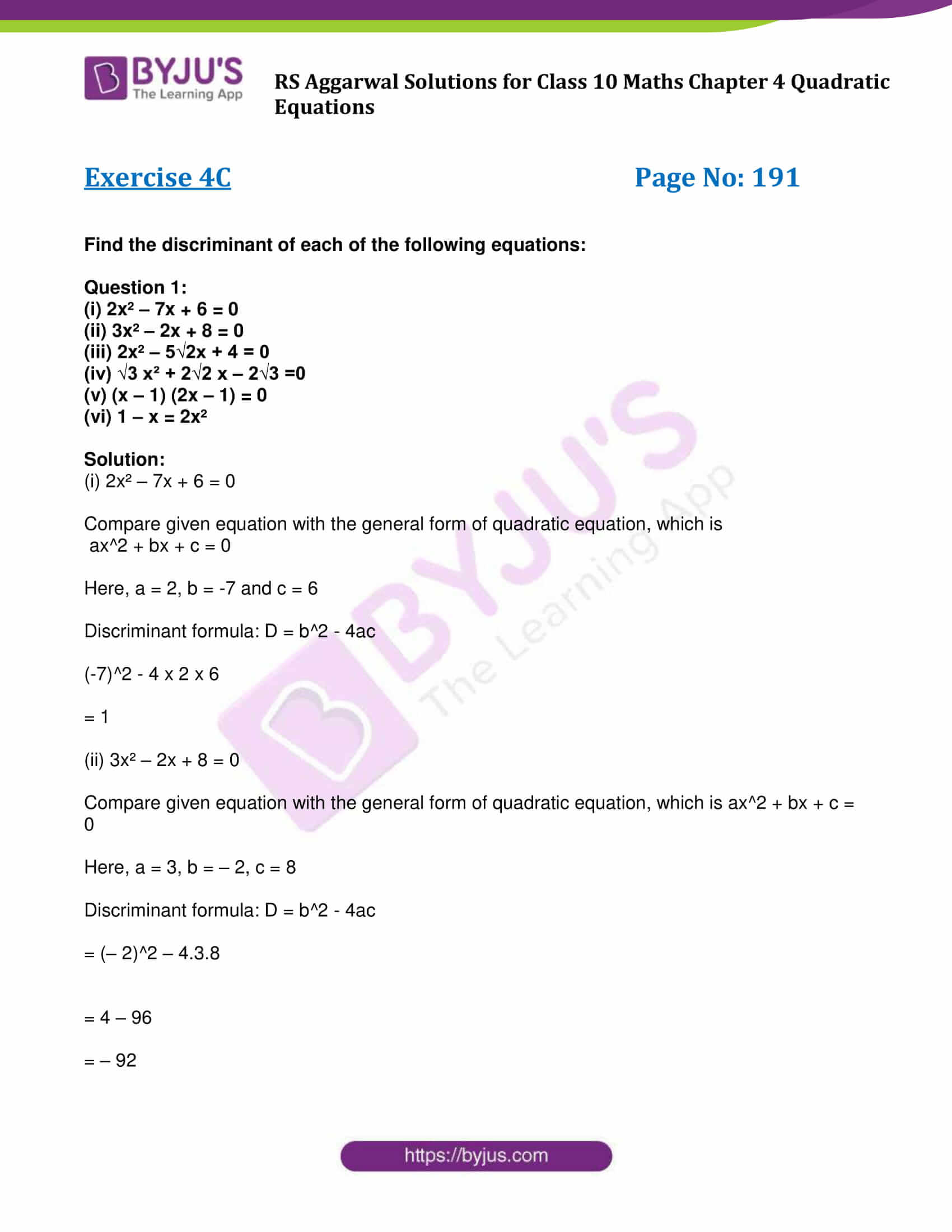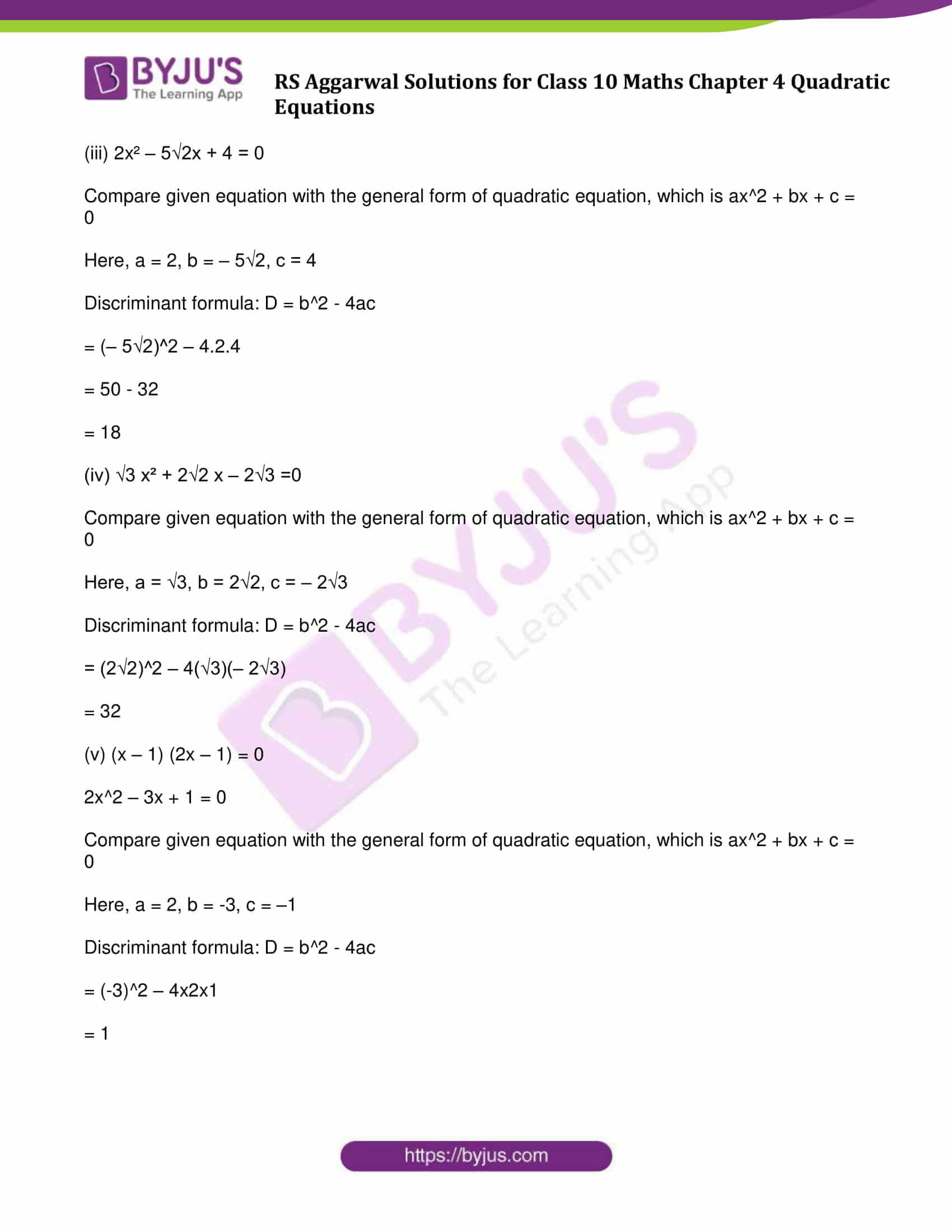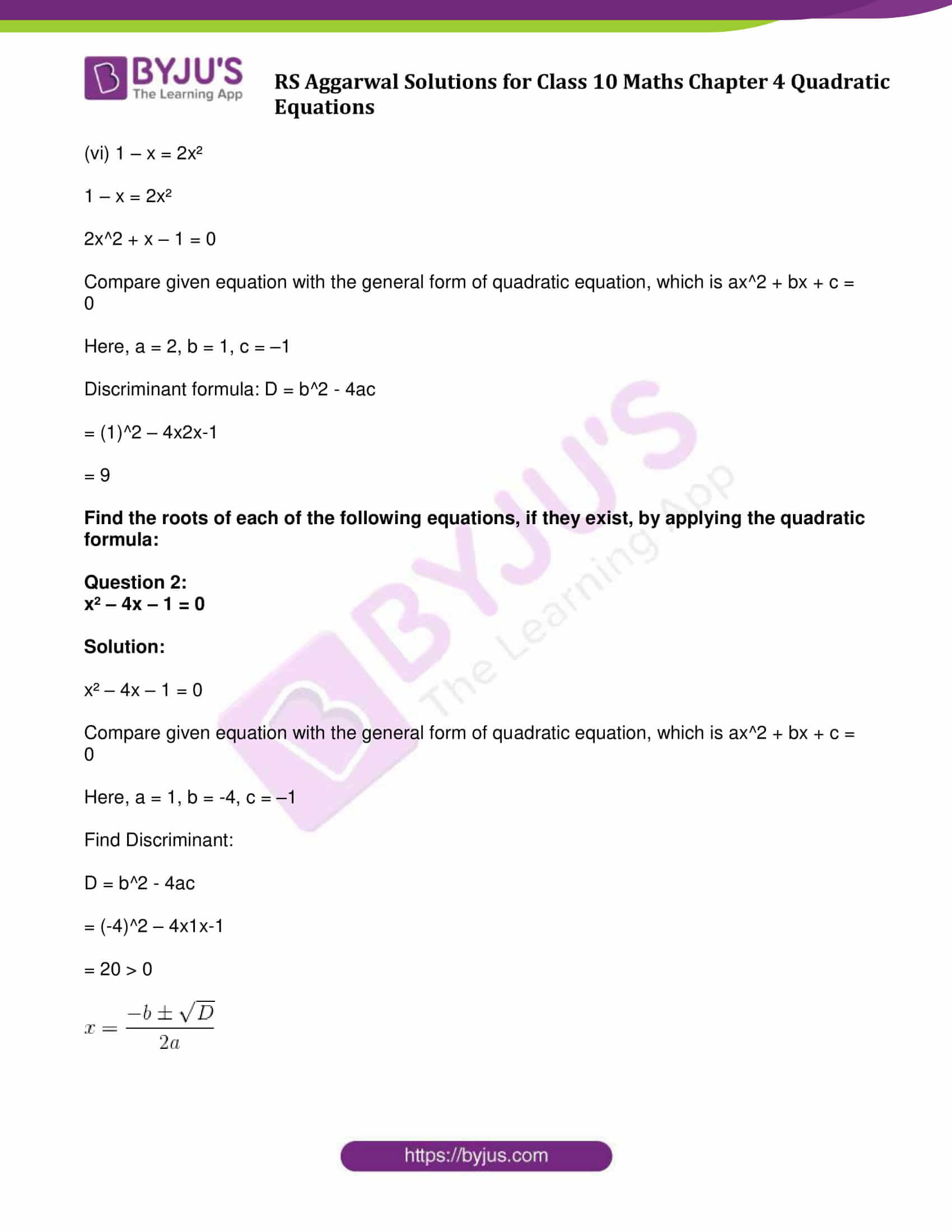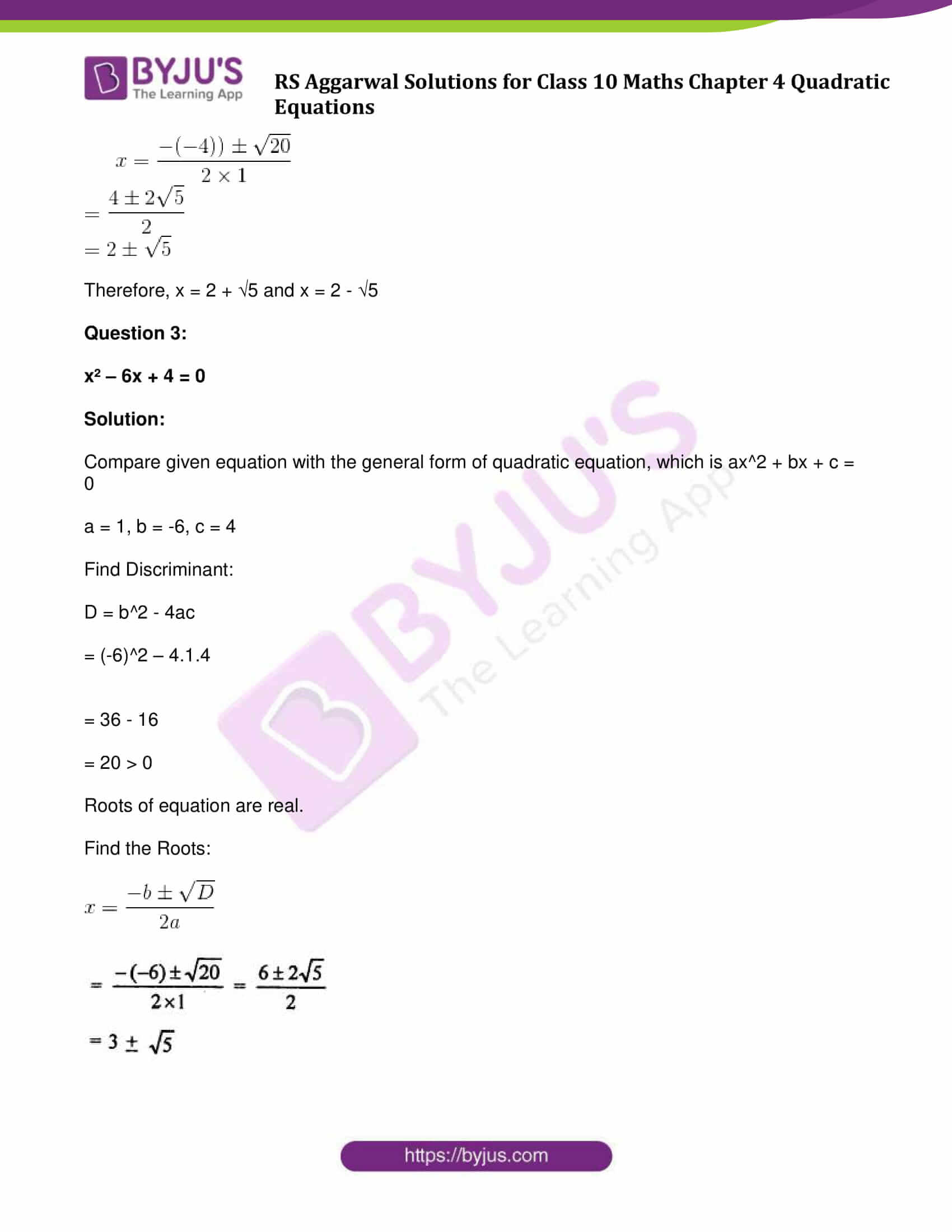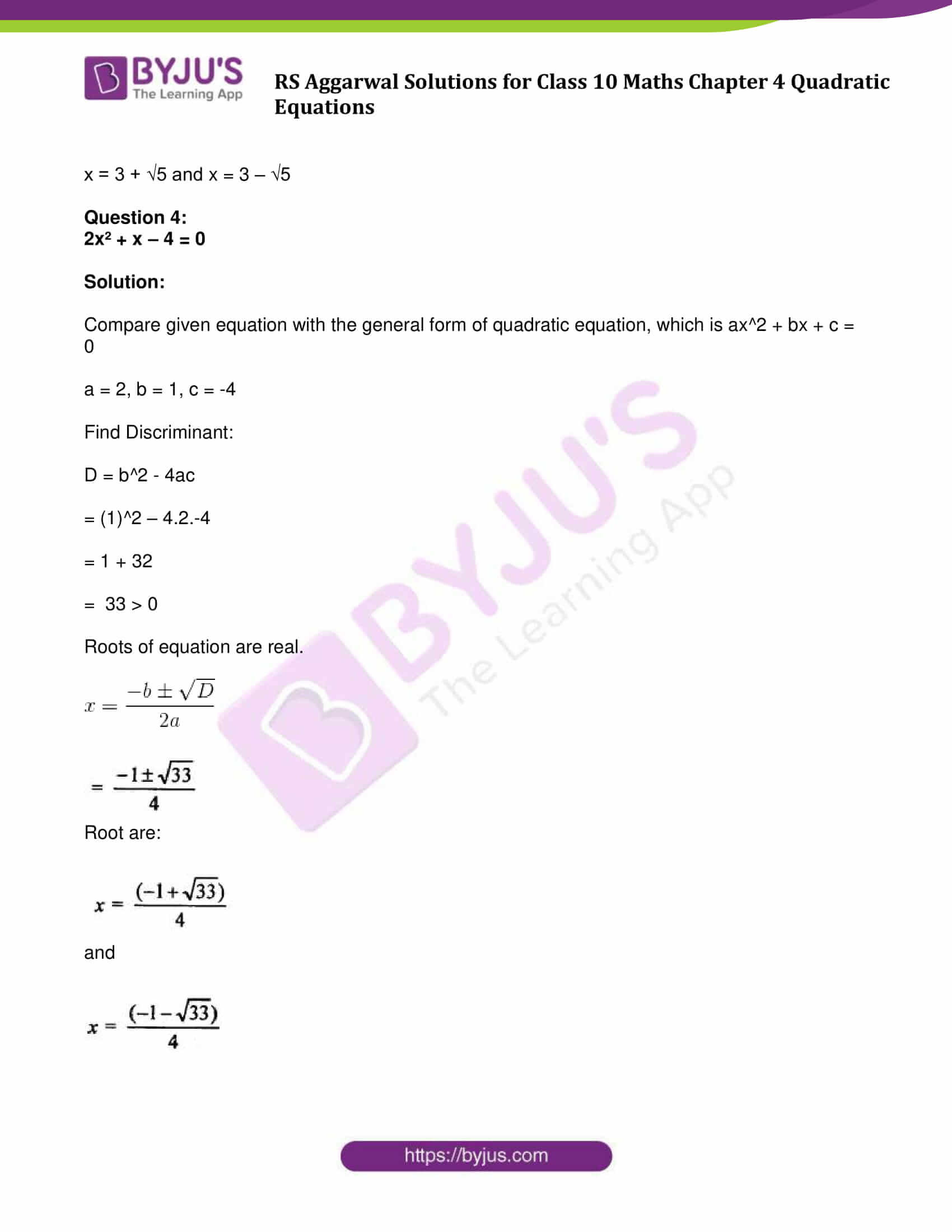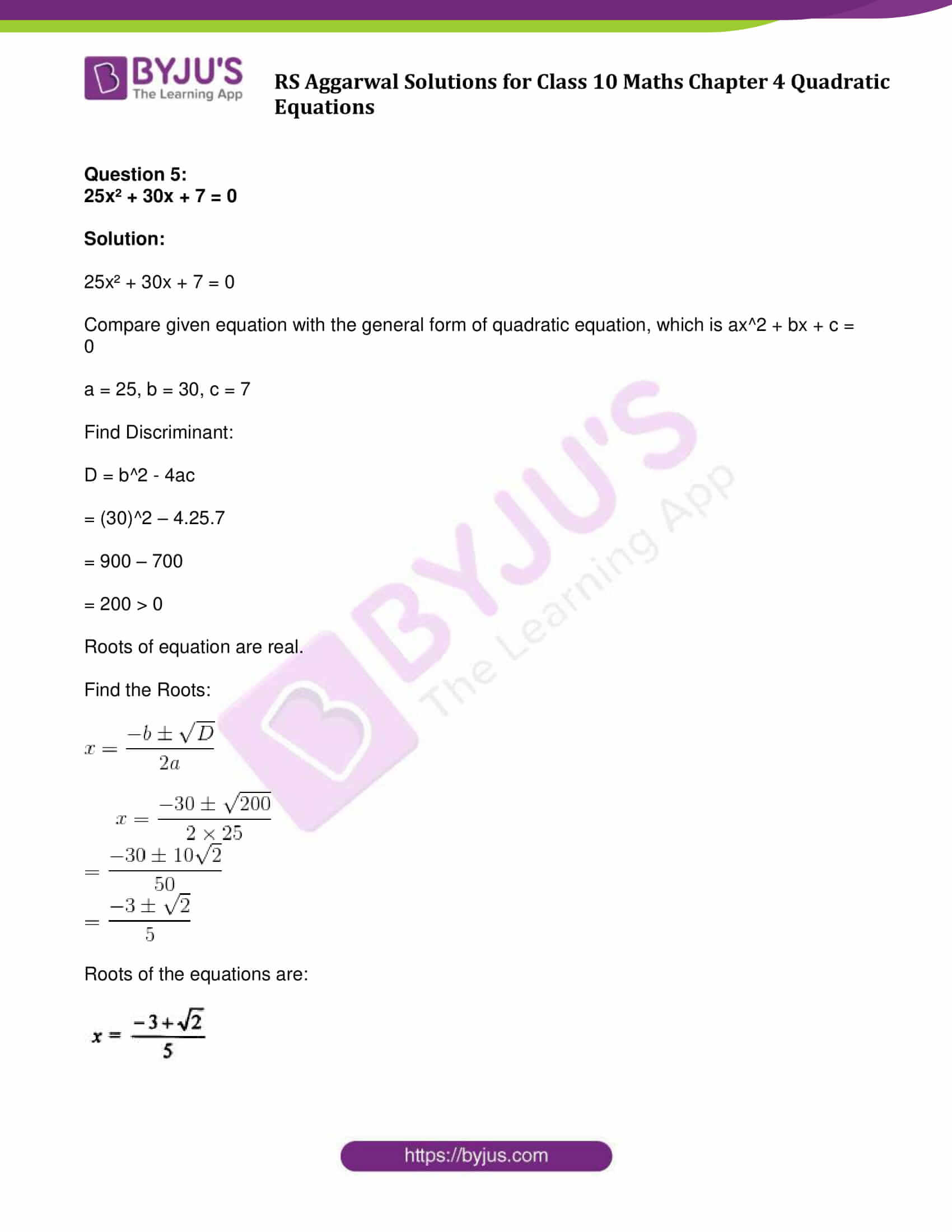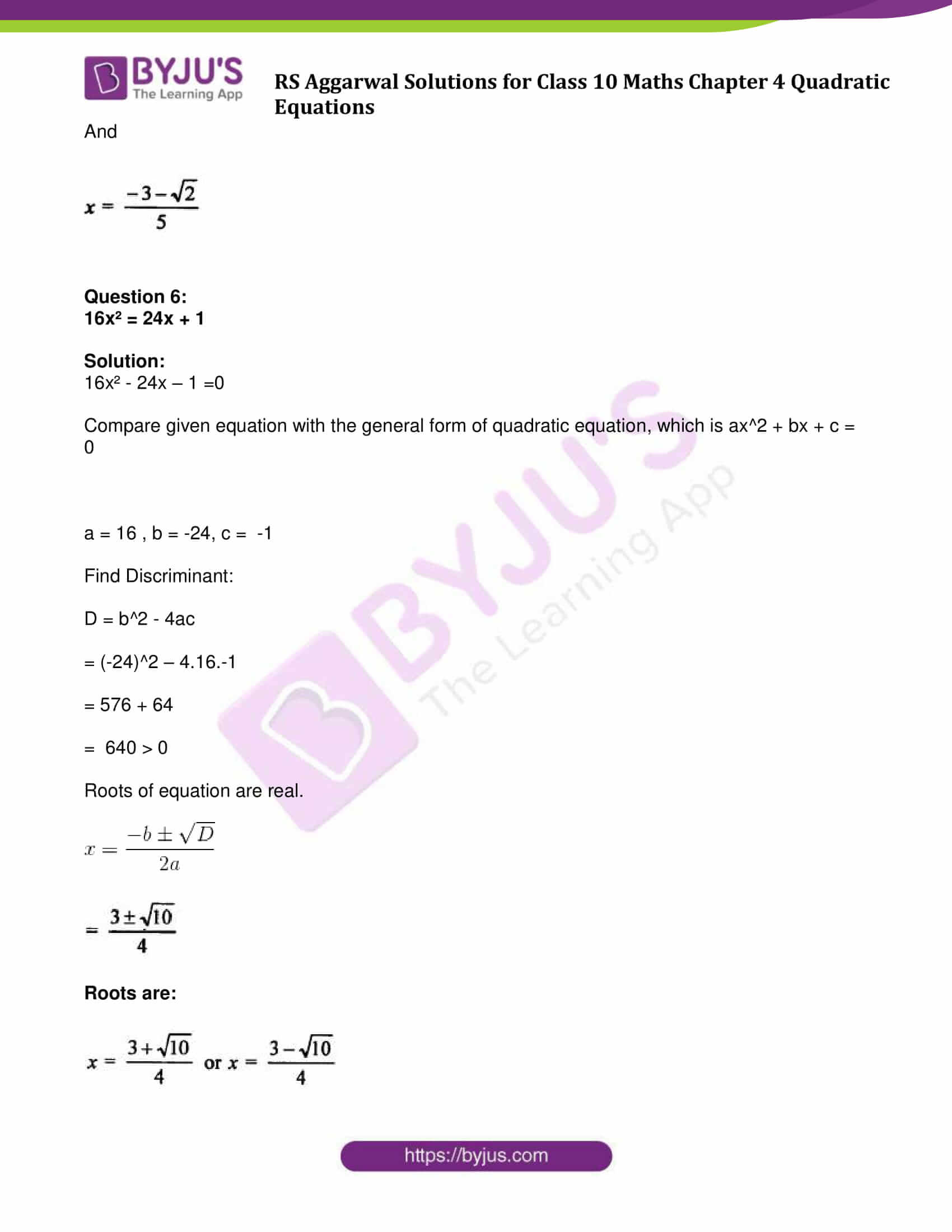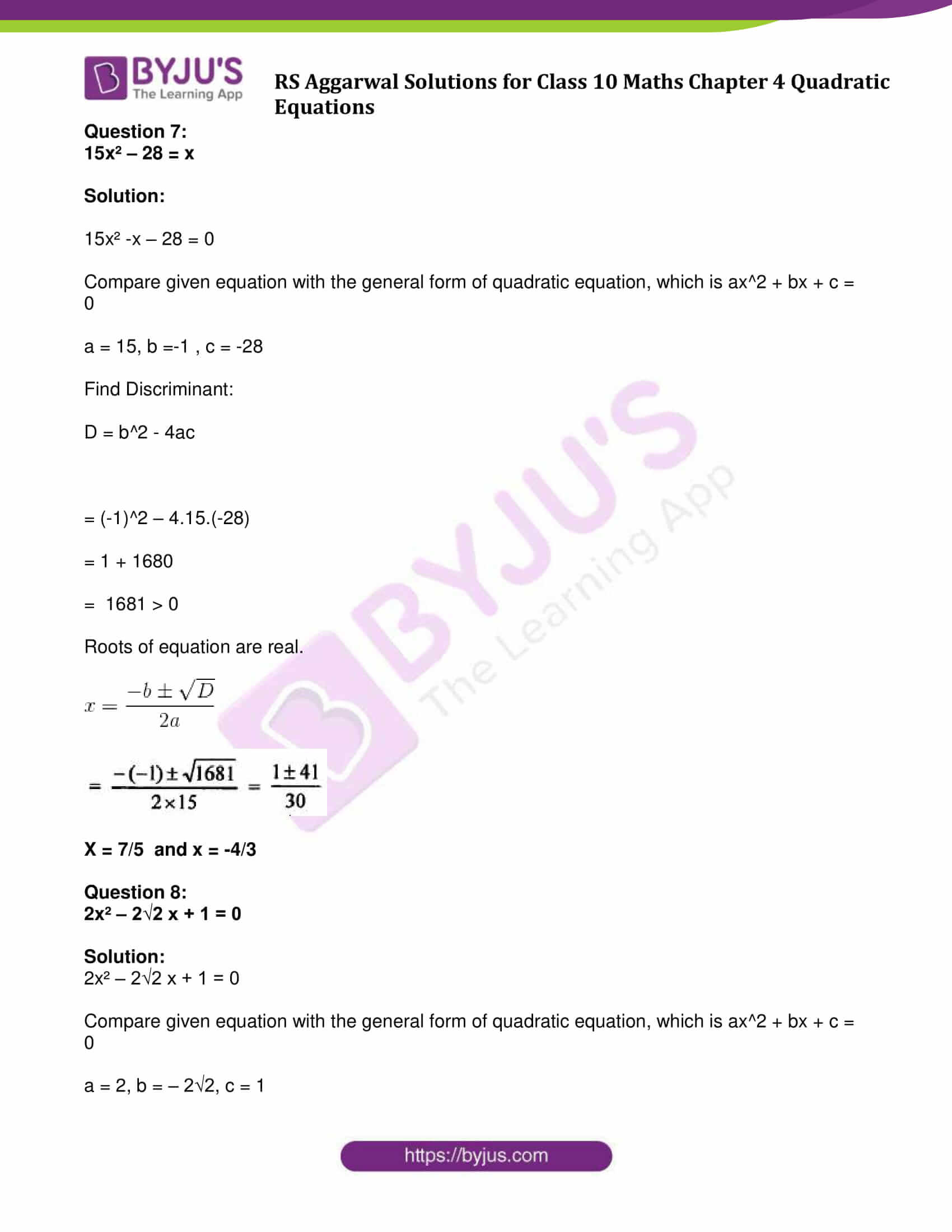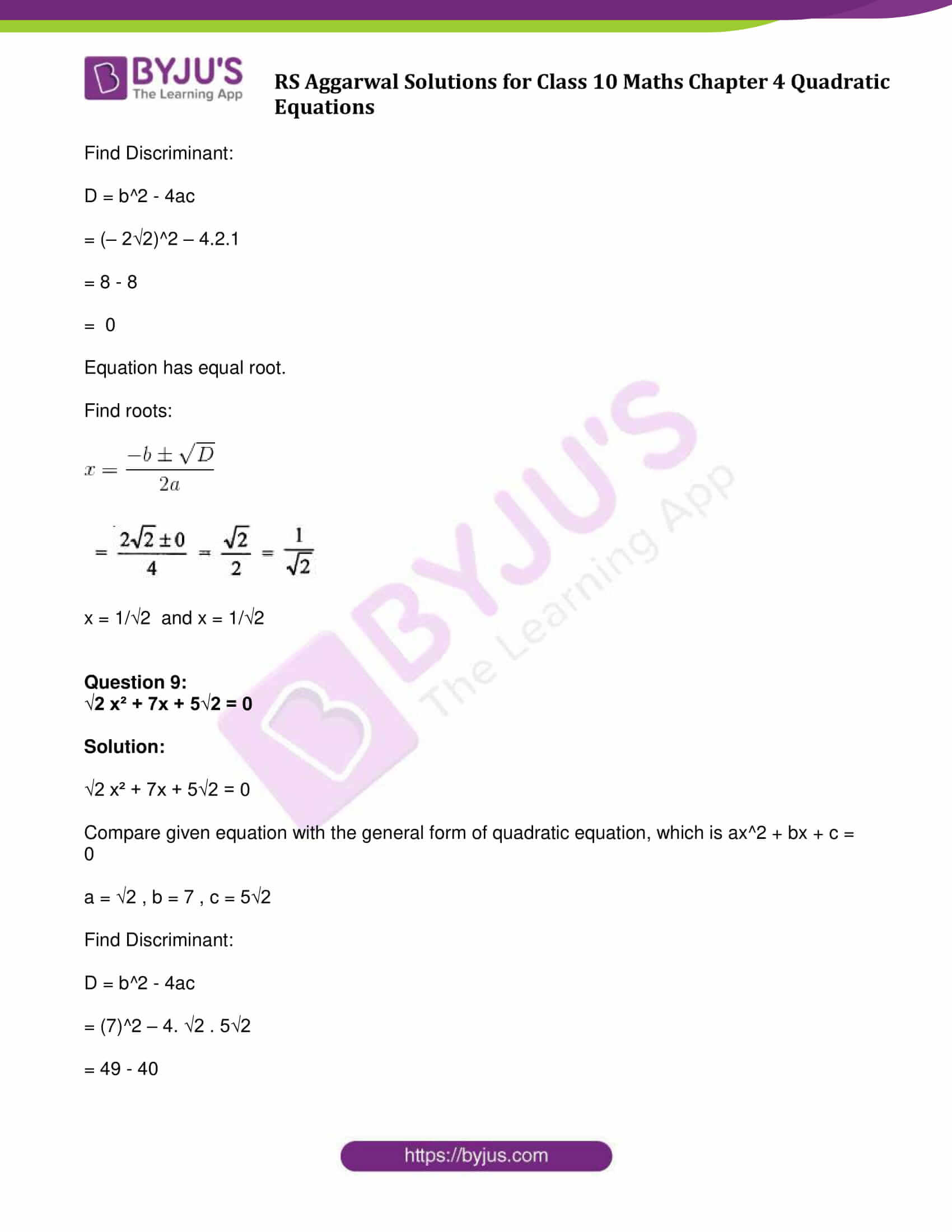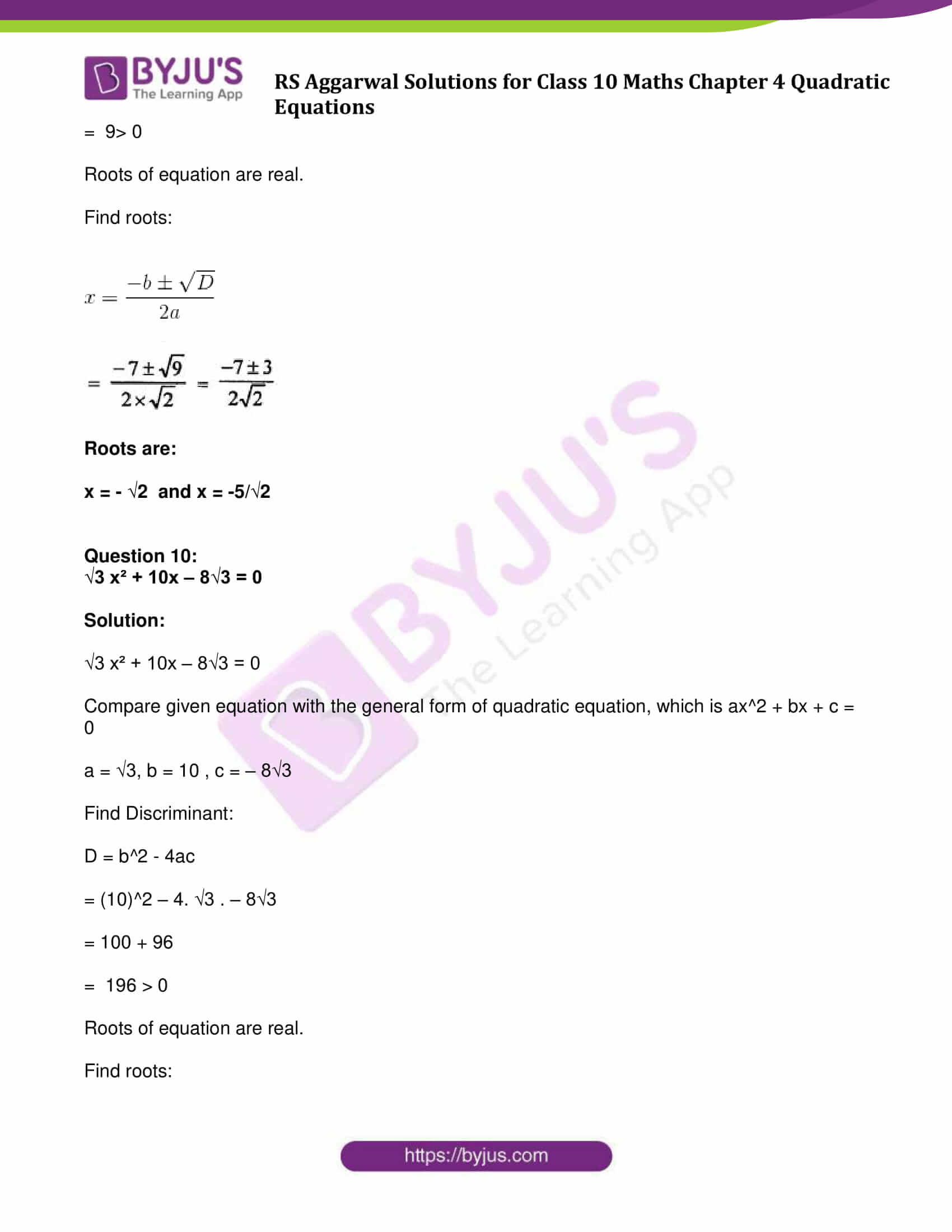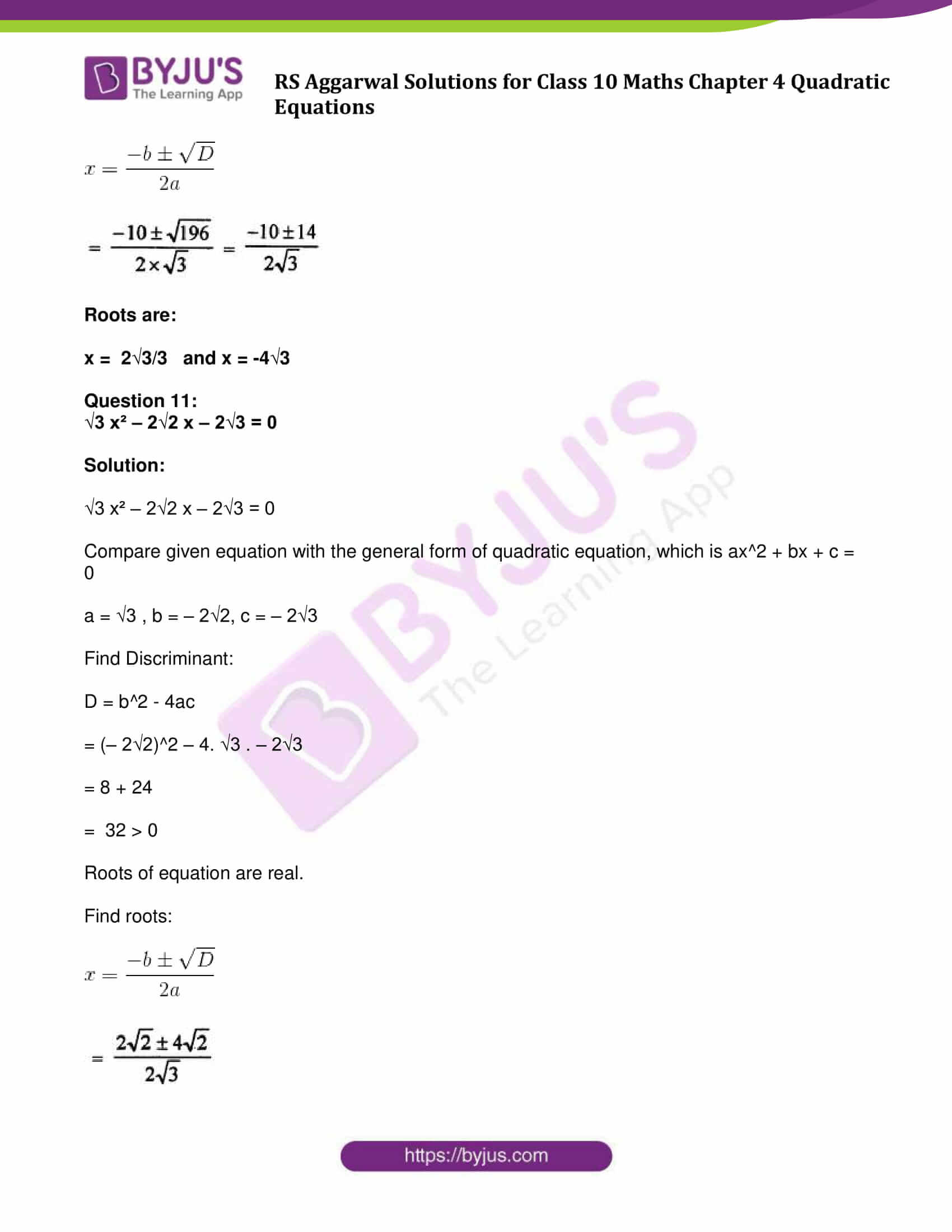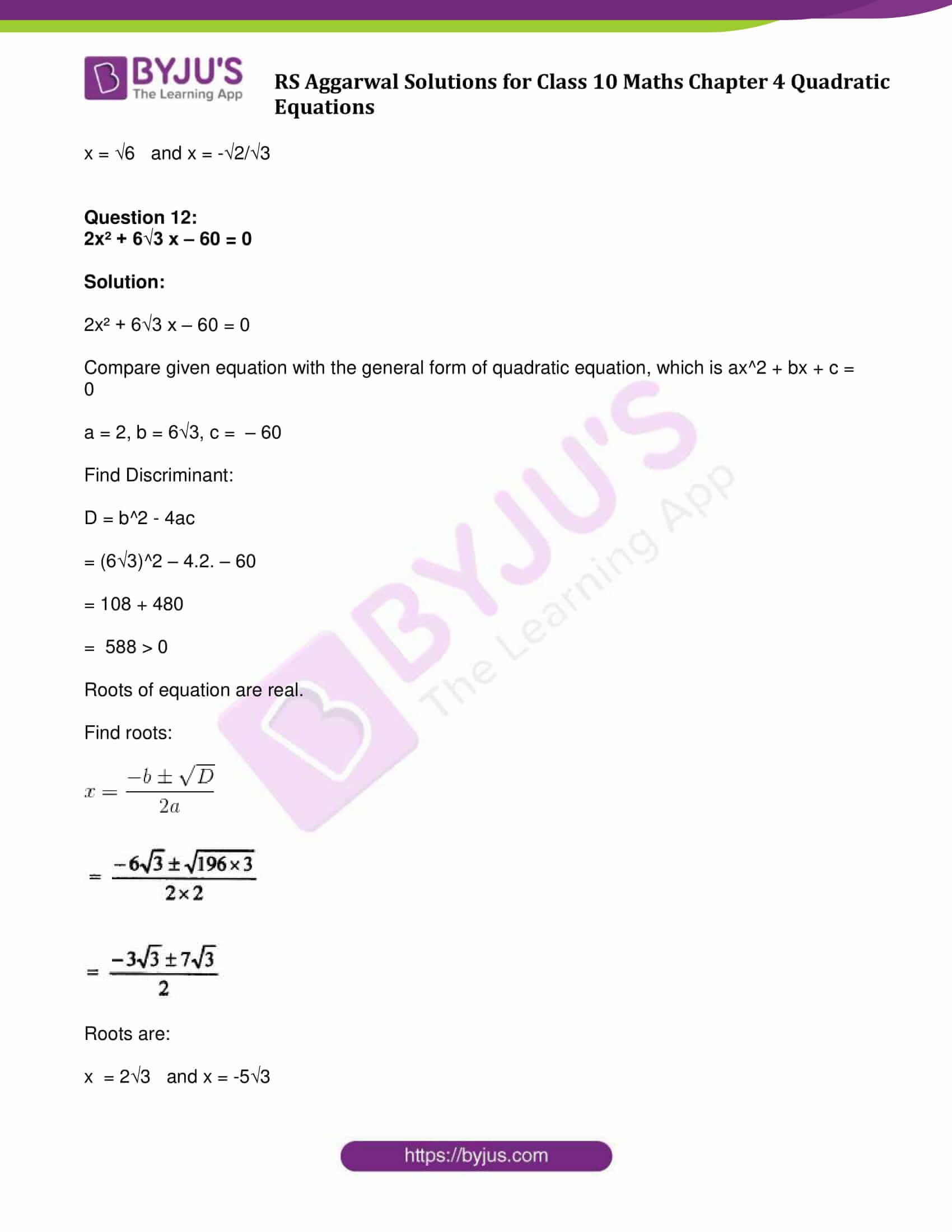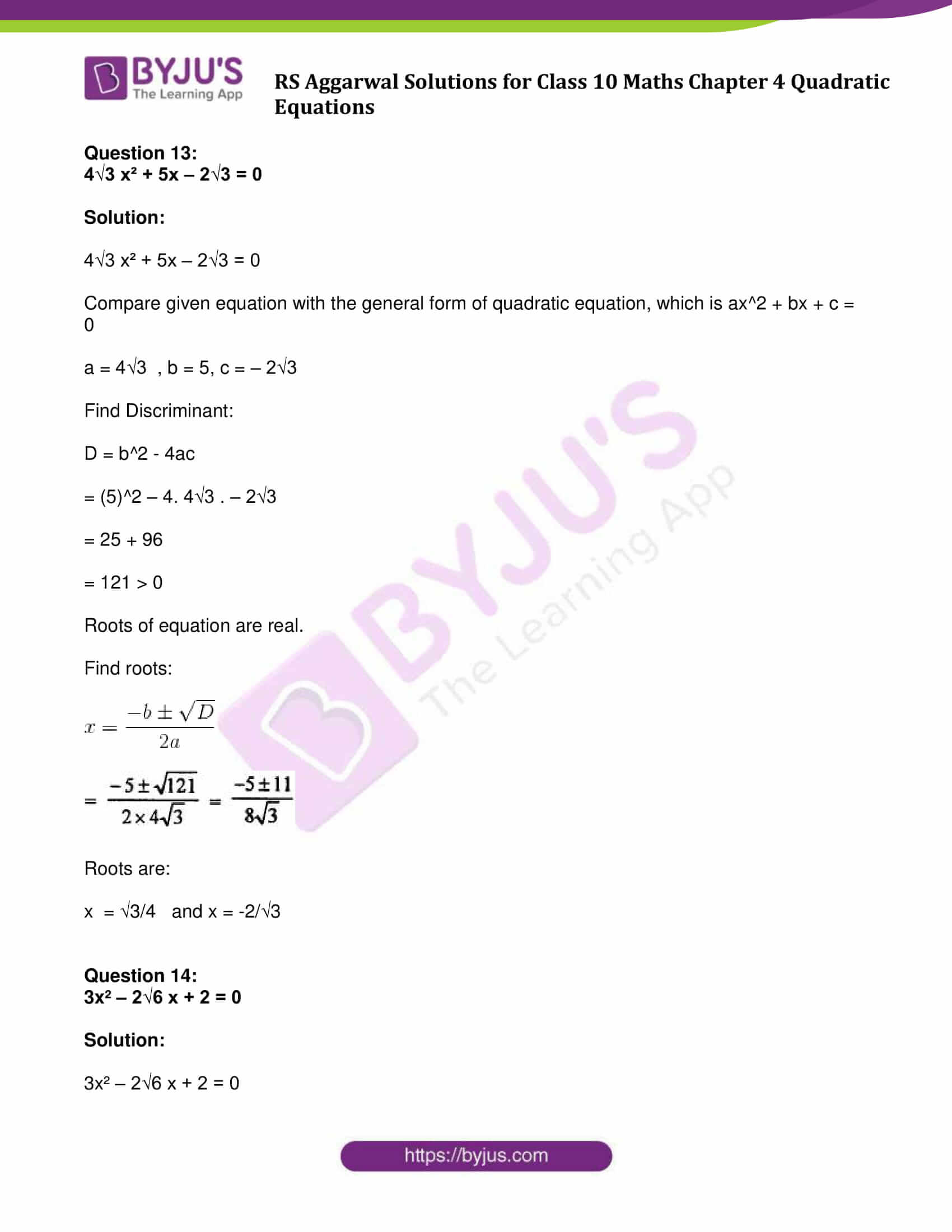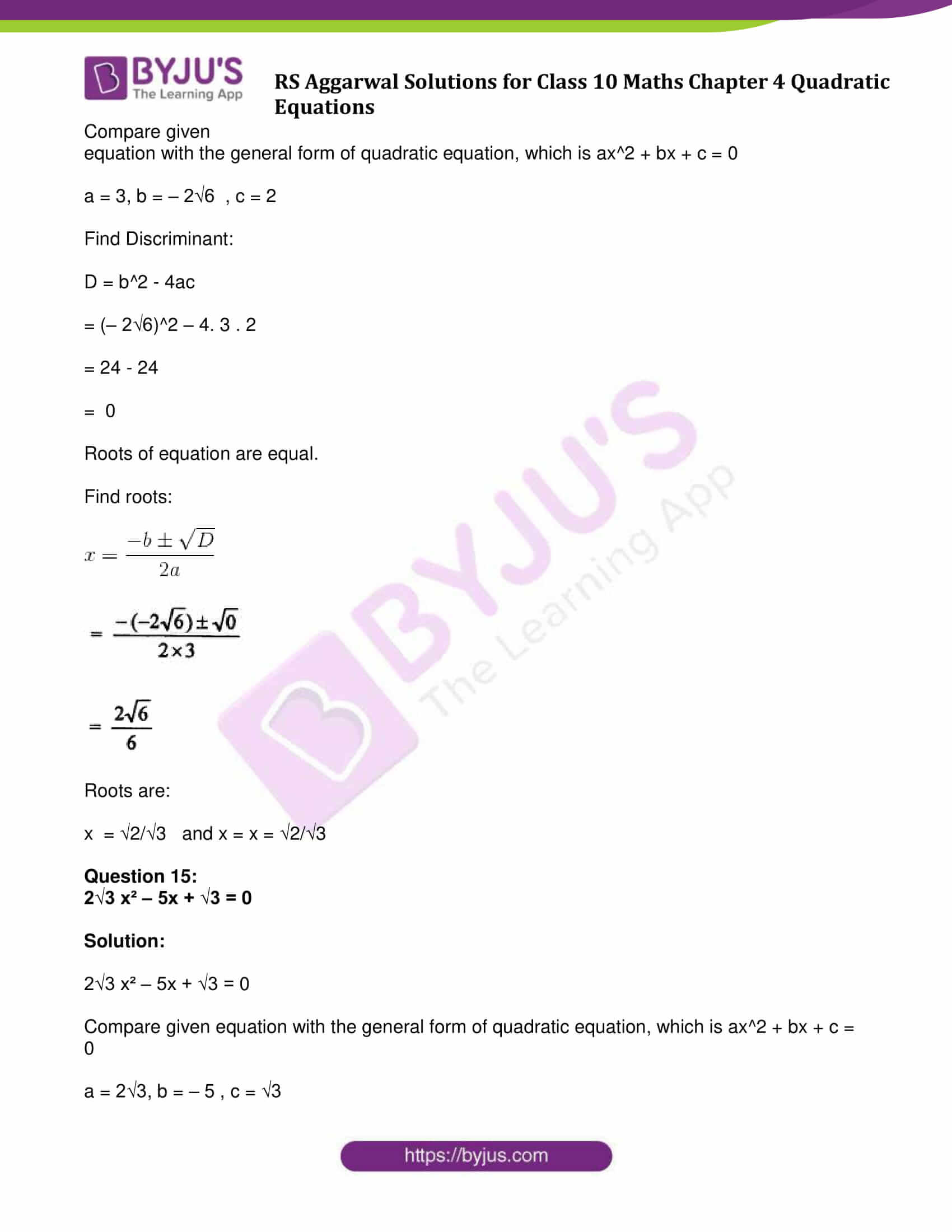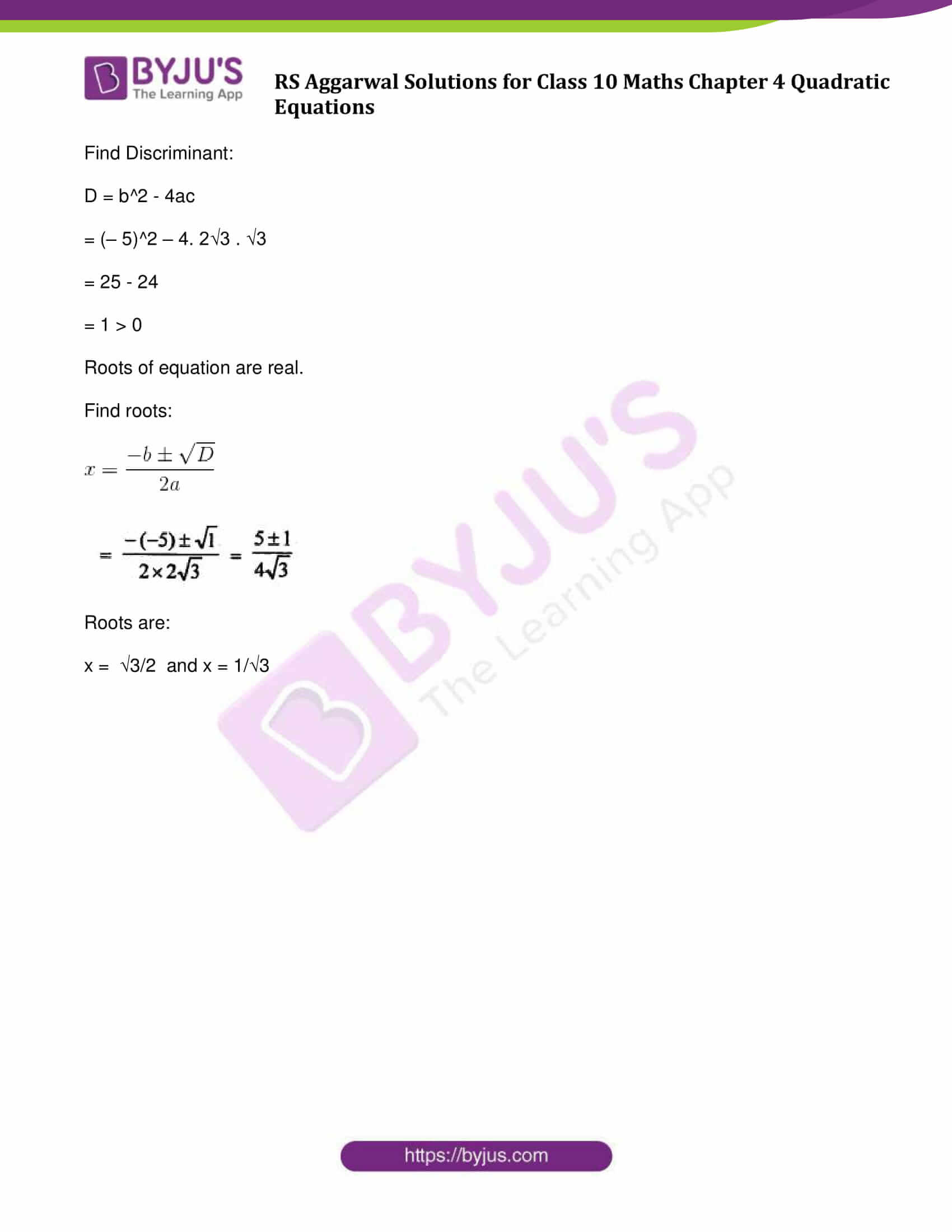### Access Other Exercise solutions of Maths R S Aggarwal Class 10 Chapter 4 – Quadratic Equations

Exercise 4A Solutions: 30 Questions (Short Answers)

Exercise 4B Solutions: 16 Questions (Short Answers)

Exercise 4D Solutions: 20 Questions (Long Answers)

## Exercise 4C Page No: 191

Find the discriminant of each of the following equations:

Question 1:

(i) 2x² – 7x + 6 = 0

(ii) 3x² – 2x + 8 = 0

(iii) 2x² – 5√2x + 4 = 0

(iv) √3 x² + 2√2 x – 2√3 =0

(v) (x – 1) (2x – 1) = 0

(vi) 1 – x = 2x²

Solution:

(i) 2x² – 7x + 6 = 0

Compare given equation with the general form of quadratic equation, which is

ax^2 + bx + c = 0

Here, a = 2, b = -7 and c = 6

Discriminant formula: D = b^2 – 4ac

(-7)^2 – 4 x 2 x 6

= 1

(ii) 3x² – 2x + 8 = 0

Compare given equation with the general form of quadratic equation, which is ax^2 + bx + c = 0

Here, a = 3, b = – 2, c = 8

Discriminant formula: D = b^2 – 4ac

= (– 2)^2 – 4.3.8

= 4 – 96

= – 92

(iii) 2x² – 5√2x + 4 = 0

Compare given equation with the general form of quadratic equation, which is ax^2 + bx + c = 0

Here, a = 2, b = – 5√2, c = 4

Discriminant formula: D = b^2 – 4ac

= (– 5√2)^2 – 4.2.4

= 50 – 32

= 18

(iv) √3 x² + 2√2 x – 2√3 =0

Compare given equation with the general form of quadratic equation, which is ax^2 + bx + c = 0

Here, a = √3, b = 2√2, c = – 2√3

Discriminant formula: D = b^2 – 4ac

= (2√2)^2 – 4(√3)(– 2√3)

= 32

(v) (x – 1) (2x – 1) = 0

2x^2 – 3x + 1 = 0

Compare given equation with the general form of quadratic equation, which is ax^2 + bx + c = 0

Here, a = 2, b = -3, c = –1

Discriminant formula: D = b^2 – 4ac

= (-3)^2 – 4x2x1

= 1

(vi) 1 – x = 2x²

1 – x = 2x²

2x^2 + x – 1 = 0

Compare given equation with the general form of quadratic equation, which is ax^2 + bx + c = 0

Here, a = 2, b = 1, c = –1

Discriminant formula: D = b^2 – 4ac

= (1)^2 – 4x2x-1

= 9

Find the roots of each of the following equations, if they exist, by applying the quadratic formula:

Question 2:

x² – 4x – 1 = 0

Solution:

x² – 4x – 1 = 0

Compare given equation with the general form of quadratic equation, which is ax^2 + bx + c = 0

Here, a = 1, b = -4, c = –1

Find Discriminant:

D = b^2 – 4ac

= (-4)^2 – 4x1x-1

= 20 > 0

$\large x = \frac{-b\pm \sqrt{D}}{2a}$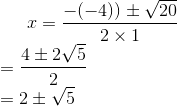Therefore, x = 2 + √5 and x = 2 – √5

Question 3:

x² – 6x + 4 = 0

Solution:

Compare given equation with the general form of quadratic equation, which is ax^2 + bx + c = 0

a = 1, b = -6, c = 4

Find Discriminant:

D = b^2 – 4ac

= (-6)^2 – 4.1.4

= 36 – 16

= 20 > 0

Roots of equation are real.

Find the Roots:

$\large x = \frac{-b\pm \sqrt{D}}{2a}$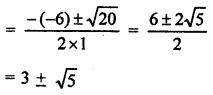x = 3 + √5 or x = 3 – √5

Question 4:

2x² + x – 4 = 0

Solution:

Compare given equation with the general form of quadratic equation, which is ax^2 + bx + c = 0

a = 2, b = 1, c = -4

Find Discriminant:

D = b^2 – 4ac

= (1)^2 – 4.2.-4

= 1 + 32

= 33 > 0

Roots of equation are real.

$\large x = \frac{-b\pm \sqrt{D}}{2a}$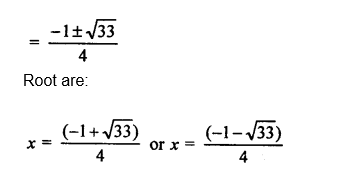Question 5:

25x² + 30x + 7 = 0

Solution:

25x² + 30x + 7 = 0

Compare given equation with the general form of quadratic equation, which is ax^2 + bx + c = 0

a = 25, b = 30, c = 7

Find Discriminant:

D = b^2 – 4ac

= (30)^2 – 4.25.7

= 900 – 700

= 200 > 0

Roots of equation are real.

Find the Roots:

$\large x = \frac{-b\pm \sqrt{D}}{2a}$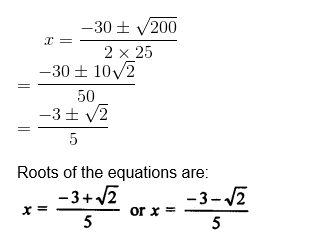Question 6:

16x² = 24x + 1

Solution:

16x² – 24x – 1 =0

Compare given equation with the general form of quadratic equation, which is ax^2 + bx + c = 0

a = 16 , b = -24, c = -1

Find Discriminant:

D = b^2 – 4ac

= (-24)^2 – 4.16.-1

= 576 + 64

= 640 > 0

Roots of equation are real.

$\large x = \frac{-b\pm \sqrt{D}}{2a}$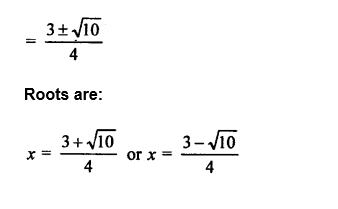Question 7:

15x² – 28 = x

Solution:

15x² -x – 28 = 0

Compare given equation with the general form of quadratic equation, which is ax^2 + bx + c = 0

a = 15, b =-1 , c = -28

Find Discriminant:

D = b^2 – 4ac

= (-1)^2 – 4.15.(-28)

= 1 + 1680

= 1681 > 0

Roots of equation are real.

$\large x = \frac{-b\pm \sqrt{D}}{2a}$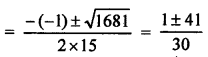X = 7/5 or x = -4/3

Question 8:

2x² – 2√2 x + 1 = 0

Solution:

2x² – 2√2 x + 1 = 0

Compare given equation with the general form of quadratic equation, which is ax^2 + bx + c = 0

a = 2, b = – 2√2, c = 1

Find Discriminant:

D = b^2 – 4ac

= (– 2√2)^2 – 4.2.1

= 8 – 8

= 0

Equation has equal root.

Find roots:

$\large x = \frac{-b\pm \sqrt{D}}{2a}$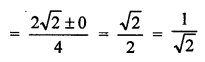x = 1/√2 or x = 1/√2

Roots of equation are real.

Question 9:

√2 x² + 7x + 5√2 = 0

Solution:

√2 x² + 7x + 5√2 = 0

Compare given equation with the general form of quadratic equation, which is ax^2 + bx + c = 0

a = √2 , b = 7 , c = 5√2

Find Discriminant:

D = b^2 – 4ac

= (7)^2 – 4. √2 . 5√2

= 49 – 40

= 9> 0

Roots of equation are real.

Find roots:

$\large x = \frac{-b\pm \sqrt{D}}{2a}$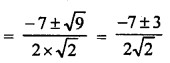Roots are:

x = – √2 or x = -5/√2

Question 10:

√3 x² + 10x – 8√3 = 0

Solution:

√3 x² + 10x – 8√3 = 0

Compare given equation with the general form of quadratic equation, which is ax^2 + bx + c = 0

a = √3, b = 10 , c = – 8√3

Find Discriminant:

D = b^2 – 4ac

= (10)^2 – 4. √3 . – 8√3

= 100 + 96

= 196 > 0

Roots of equation are real.

Find roots:

$\large x = \frac{-b\pm \sqrt{D}}{2a}$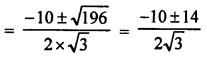Roots are:

x = 2√3/3 or x = -4√3

Question 11:

√3 x² – 2√2 x – 2√3 = 0

Solution:

√3 x² – 2√2 x – 2√3 = 0

Compare given equation with the general form of quadratic equation, which is ax^2 + bx + c = 0

a = √3 , b = – 2√2, c = – 2√3

Find Discriminant:

D = b^2 – 4ac

= (– 2√2)^2 – 4. √3 . – 2√3

= 8 + 24

= 32 > 0

Roots of equation are real.

Find roots:

$\large x = \frac{-b\pm \sqrt{D}}{2a}$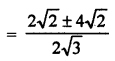x = √6 or x = -√2/√3

Question 12:

2x² + 6√3 x – 60 = 0

Solution:

2x² + 6√3 x – 60 = 0

Compare given equation with the general form of quadratic equation, which is ax^2 + bx + c = 0

a = 2, b = 6√3, c = – 60

Find Discriminant:

D = b^2 – 4ac

= (6√3)^2 – 4.2. – 60

= 108 + 480

= 588 > 0

Roots of equation are real.

Find roots:

$\large x = \frac{-b\pm \sqrt{D}}{2a}$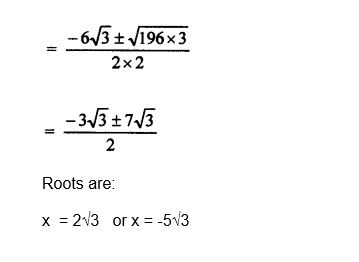Question 13:

4√3 x² + 5x – 2√3 = 0

Solution:

4√3 x² + 5x – 2√3 = 0

Compare given equation with the general form of quadratic equation, which is ax^2 + bx + c = 0

a = 4√3 , b = 5, c = – 2√3

Find Discriminant:

D = b^2 – 4ac

= (5)^2 – 4. 4√3 . – 2√3

= 25 + 96

= 121 > 0

Roots of equation are real.

Find roots:

$\large x = \frac{-b\pm \sqrt{D}}{2a}$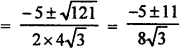Roots are:

x = √3/4 or x = -2/√3

Question 14:

3x² – 2√6 x + 2 = 0

Solution:

3x² – 2√6 x + 2 = 0

Compare given equation with the general form of quadratic equation, which is ax^2 + bx + c = 0

a = 3, b = – 2√6 , c = 2

Find Discriminant:

D = b^2 – 4ac

= (– 2√6)^2 – 4. 3 . 2

= 24 – 24

= 0

Roots of equation are equal.

Find roots:

$\large x = \frac{-b\pm \sqrt{D}}{2a}$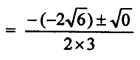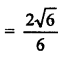Roots are:

x = √2/√3 and x = x = √2/√3

Question 15:

2√3 x² – 5x + √3 = 0

Solution:

2√3 x² – 5x + √3 = 0

Compare given equation with the general form of quadratic equation, which is ax^2 + bx + c = 0

a = 2√3, b = – 5 , c = √3

Find Discriminant:

D = b^2 – 4ac

= (– 5)^2 – 4. 2√3 . √3

= 25 – 24

= 1 > 0

Roots of equation are real.

Find roots:

$\large x = \frac{-b\pm \sqrt{D}}{2a}$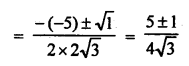Roots are:

x = √3/2 and x = 1/√3

## R S Aggarwal Solutions for Class 10 Maths Chapter 4 Quadratic Equations Exercise 4C

Class 10 Maths Chapter 4 Quadratic Equations Exercise 4C is based on the topics: